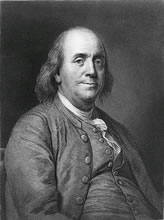# Benjamin Franklin’s Fluid Theory

Benjamin Franklin (1750) imagined the electricity as an invisible fluid. Franklin assured that if anybody had more fluid than usual, it could have a positive charge, but if it had less fluid than normal it had negative charge. Based on that, the assumption that the electricity flows from the positive to the negative came out.

Benjamin Franklin’s Fluid Theory was easy to understand and it was accepted as correct. This theory agreed with the experiments that were carried out on the 18 and 19 centuries.

Everybody accepted the idea that charges flows from the positive to the negative (conventional flow) and it was accepted as the truth. It emerged numerous concepts and formulas based on the conventional flow of the current between years 1750 and 1897.Later it was discovered that the flow of the current was not running from the positive to the negative, but from the negative to the positive. (We have to remember that the current is a flow of electrons, that are negatively charged)

However, since this current is a flow of electrons, we can say that it is a negative current. When this flow is running throughout the conductor, it leaves a hole which is traveling from the opposite way of the flow, then we say that this current is positive. That explains the assumption made by Benjamin Franklin.

Therefore, Benjamin Franklin’s Fluid Theory is not true, though it works as if it were true.

•
•
• 1
•
•
•
•
•
1
Share
•
1
Share
•
•
• 1
•
•
•
•